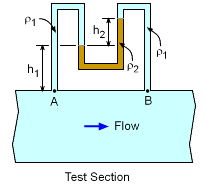Ch 2. Fluid Statics Multimedia Engineering Fluids PressureVariation PressureMeasurement HydrostaticForce(Plane) HydrostaticForce(Curved) Buoyancy
 Chapter 1. Basics 2. Fluid Statics 3. Kinematics 4. Laws (Integral) 5. Laws (Diff.) 6. Modeling/Similitude 7. Inviscid 8. Viscous 9. External Flow 10. Open-Channel Appendix Basic Math Units Basic Equations Water/Air Tables Sections Search eBooks Dynamics Fluids Math Mechanics Statics Thermodynamics Author(s): Chean Chin Ngo Kurt Gramoll ©Kurt GramollFLUID MECHANICS - CASE STUDY SOLUTIONU-Tube Differential Manometer To determine the pressure difference between point A and B, start from one end and work to the other end:      pA - ρ1gh1 - ρ2gh2 + ρ1g (h1 + h2) = pB Rearrange terms to yield:      pA - pB = gh2 (ρ2 - ρ1) For the given values of ρ1 = 1000 kg/m3, ρ2 = 1600 kg/m3 and h2 = 0.75 m:      pA - pB = (9.8 m/s2) (0.75 m) (1600 - 1000) kg/m3                  = 4.4 kPa

Practice Homework and Test problems now available in the 'Eng Fluids' mobile app
Includes over 250 problems with complete detailed solutions.
Available now at the Google Play Store and Apple App Store.